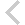FICO Xpress Optimization Examples Repository
 FICO Optimization Community FICO Xpress Optimization HomeIntroductory examples

Description
approx burglar chess Problem name and type, features Difficulty Approximation: Piecewise linear approximation ** SOS-2, Special Ordered Sets, piecewise linear approximation of a nonlinear function MIP modeling: Knapsack problem: 'Burglar' * simple MIP model with binary variables, data input from text data file, array initialization, numerical indices, string indices, record data structure LP modeling: Production planning: 'Chess' problem * simple LP model, solution output, primal solution values, slack values, activity values, dual solution values All item discount pricing: Piecewise linear function *** SOS-1, Special Ordered Sets, piecewise linear function, approximation of non-continuous function, step function Incremental pricebreaks: Piecewise linear function *** SOS-2, Special Ordered Sets, piecewise linear function, step function

Further explanation of this example: 'Applications of optimization with Xpress-MP', Introductory examples (Chapters 1 to 5) of the book 'Applications of optimization with Xpress-MP'

Source Files

Data Files

pricebrinc2.mos

```(!******************************************************
Mosel Example Problems
======================

file pricebrinc2.mos
````````````````````
Incremental pricebreaks formulated with
binary variables

(c) 2008 Fair Isaac Corporation
author: S. Heipcke, Sep. 2006
*******************************************************!)

model "Incremental pricebreaks (binaries)"
uses "mmxprs"

declarations
NB = 3                                ! Number of price bands
BREAKS = 1..NB
COST: array(BREAKS) of real           ! Cost per unit
x: array(BREAKS) of mpvar             ! Number of items bought at a price
b: array(BREAKS) of mpvar             ! Indicators of price bands
B: array(0..NB) of real               ! Break points of cost function
end-declarations

DEM:= 150                              ! Demand
B::  [0, 50, 120, 200]
COST:: [0.8, 0.5, 0.3]

forall(i in BREAKS) b(i) is_binary

! Objective: total price
TotalCost:= sum(i in BREAKS) COST(i)*x(i)

! Meet the demand
sum(i in BREAKS) x(i) = DEM

! Lower and upper bounds on quantities
forall(i in 1..NB-1) (B(i)-B(i-1)) * b(i+1) <= x(i)
forall(i in BREAKS)  x(i) <= (B(i)-B(i-1)) * b(i)

! Sequence of price intervals
forall(i in 1..NB-1) b(i) >= b(i+1)

! Solve the problem
minimize(TotalCost)

! Solution printing
writeln("Objective: ", getobjval,
" (price per unit: ", getobjval/DEM, ")")
forall(i in BREAKS)
writeln("x(", i, "): ", getsol(x(i)), " (price per unit: ", COST(i), ")")

end-model

```